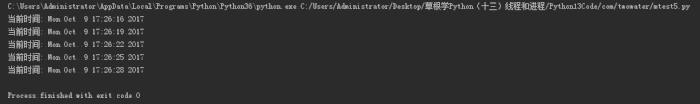# 进程

Python 中的多线程其实并不是真正的多线程，如果想要充分地使用多核 CPU 的资源，在 Python 中大部分情况需要使用多进程。

Python 提供了非常好用的多进程包 multiprocessing，只需要定义一个函数，Python 会完成其他所有事情。

## 1、类 Process

• target 表示调用对象
• args 表示调用对象的位置参数元组
• kwargs表示调用对象的字典
• name为别名
• group实质上不使用

``````#!/usr/bin/env python3
# -*- coding: UTF-8 -*-

import multiprocessing
import time

def worker(interval, name):
print(name + '【start】')
time.sleep(interval)
print(name + '【end】')

if __name__ == "__main__":
p1 = multiprocessing.Process(target=worker, args=(2, '两点水1'))
p2 = multiprocessing.Process(target=worker, args=(3, '两点水2'))
p3 = multiprocessing.Process(target=worker, args=(4, '两点水3'))

p1.start()
p2.start()
p3.start()

print("The number of CPU is:" + str(multiprocessing.cpu_count()))
for p in multiprocessing.active_children():
print("child   p.name:" + p.name + "\tp.id" + str(p.pid))
print("END!!!!!!!!!!!!!!!!!")

``````## 2、把进程创建成类

``````# -*- coding: UTF-8 -*-

import multiprocessing
import time

class ClockProcess(multiprocessing.Process):
def __init__(self, interval):
multiprocessing.Process.__init__(self)
self.interval = interval

def run(self):
n = 5
while n > 0:
print("当前时间: {0}".format(time.ctime()))
time.sleep(self.interval)
n -= 1

if __name__ == '__main__':
p = ClockProcess(3)
p.start()

``````## 3、daemon 属性

``````# -*- coding: UTF-8 -*-
import multiprocessing
import time

def worker(interval):
print('工作开始时间：{0}'.format(time.ctime()))
time.sleep(interval)
print('工作结果时间：{0}'.format(time.ctime()))

if __name__ == '__main__':
p = multiprocessing.Process(target=worker, args=(3,))
p.start()
print('【EMD】')

``````

``````【EMD】

``````

``````# -*- coding: UTF-8 -*-

import multiprocessing
import time

def worker(interval):
print('工作开始时间：{0}'.format(time.ctime()))
time.sleep(interval)
print('工作结果时间：{0}'.format(time.ctime()))

if __name__ == '__main__':
p = multiprocessing.Process(target=worker, args=(3,))
p.daemon = True
p.start()
print('【EMD】')
``````

``````【EMD】
``````

## 4、join 方法

``````import multiprocessing
import time

def worker(interval):
print('工作开始时间：{0}'.format(time.ctime()))
time.sleep(interval)
print('工作结果时间：{0}'.format(time.ctime()))

if __name__ == '__main__':
p = multiprocessing.Process(target=worker, args=(3,))
p.daemon = True
p.start()
p.join()
print('【EMD】')
``````

``````工作开始时间：Tue Oct 10 11:30:08 2017

【EMD】
``````

## 5、Pool

``````# -*- coding: UTF-8 -*-

from multiprocessing import Pool
import os, time, random

def long_time_task(name):
print('进程的名称：{0} ；进程的PID: {1} '.format(name, os.getpid()))
start = time.time()
time.sleep(random.random() * 3)
end = time.time()
print('进程 {0} 运行了 {1} 秒'.format(name, (end - start)))

if __name__ == '__main__':
print('主进程的 PID：{0}'.format(os.getpid()))
p = Pool(4)
for i in range(6):
p.apply_async(long_time_task, args=(i,))
p.close()
# 等待所有子进程结束后在关闭主进程
p.join()
print('【End】')
``````

``````主进程的 PID：7256

【End】
``````

``````p = Pool(5)
``````

## 6、进程间通信

Process 之间肯定是需要通信的，操作系统提供了很多机制来实现进程间的通信。Python 的 multiprocessing 模块包装了底层的机制，提供了Queue、Pipes 等多种方式来交换数据。

``````#!/usr/bin/env python3
# -*- coding: UTF-8 -*-

from multiprocessing import Process, Queue
import os, time, random

def write(q):
# 写数据进程
print('写进程的PID:{0}'.format(os.getpid()))
for value in ['两点水', '三点水', '四点水']:
print('写进 Queue 的值为：{0}'.format(value))
q.put(value)
time.sleep(random.random())

def read(q):
# 读取数据进程
print('读进程的PID:{0}'.format(os.getpid()))
while True:
value = q.get(True)
print('从 Queue 读取的值为：{0}'.format(value))

if __name__ == '__main__':
# 父进程创建 Queue，并传给各个子进程
q = Queue()
pw = Process(target=write, args=(q,))
pr = Process(target=read, args=(q,))
# 启动子进程 pw
pw.start()
# 启动子进程pr
pr.start()
# 等待pw结束:
pw.join()
# pr 进程里是死循环，无法等待其结束，只能强行终止
pr.terminate()

``````

``````读进程的PID:13208

``````